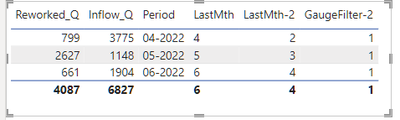cancel
Showing results for
Did you mean:

Fabric is Generally Available. Browse Fabric Presentations. Work towards your Fabric certification with the Cloud Skills Challenge.Post Prodigy

## Can you make a gauge variable?

So I build this gauge:Now it (of course) reacts when I set the slicer to another quarter.

My idea was now to have 1 big gauge for the quarter and make 3 little ones below it for the separate months.

How would you make that work?

Yes in the small ones you could set the month in the filter for all 3.

But of course when I select then Q4 the months of Q4 should be selected in the 3 small ones and

not the ones (fixed) for Q3.

Can it be done?

5 REPLIES 5Post Prodigy

Ok guys i'm giving up....it is really not worth the anger 😂

Either it cannot be done in Power BI or my data (which is fairly simple) is still not suited for it.

Thought my last shot would work but....no.Really thought it would not be that difficult. 1 big gauge that gives the Quarter numbers (in this case Q3).

Then 3 smaller gauges below to give the months.

Had it all worked out in a table as you see.

MaxMth = CALCULATE(MAX(Dim_Date[Month Number]),ALLSELECTED())
MidMth = CALCULATE(AVERAGE(Dim_Date[Month Number]),ALLSELECTED())
MinMth = CALCULATE(MIN(Dim_Date[Month Number]),ALLSELECTED())

So now I thought I could make a sort of filter with Switch like:
If month number = MaxMth then "H", if month number = MidMth then "M" and if month number = MinMth then "L"

But somehow it cannot get this in a filter. Wanted to end up with a filter that give H, M and L as option.
Then for the 3 smaller gauges I can hardcode the filter to H, M or L.
Seemed like a good solution....but I keep missing the last part in all my solutions 😣

As said I'm done.....not worth all the angerPost Prodigy

Ok still not working but maybe I found the issue.

I think my measures are not working correctly.

I now for better visibility put it for now in a tabel and Quarter 2 is selected :As you see last month should be 6. So also for April and May it shoud show 6.

Of course as a result LastMth - 2 will also be incorrect because that should be 4 everywhere.

And then het GaugeFilter-2 would only show a 1 for the line of April (4) and the two later months should be 0.

Could somebody help me adjust my measures?

LastMth =
FORMAT(CALCULATE(MAX(Dim_Date[Date]),FILTER('AAA Rework Merge',ABS(SUM('AAA Rework Merge'[Quantity]))>0)),
"M")

LastMth-2 =
FORMAT(CALCULATE(MAX(Dim_Date[Date]),FILTER('AAA Rework Merge',ABS(SUM('AAA Rework Merge'[Quantity]))>0)),
"M") -2

GaugeFilter-2 =
IF([LastMth] -2 = [LastMth-2], 1, 0)

*Editt :  Important to tell here is that when you take out the field period all looks fine.Post Prodigy

I feel I am being close with this.

I made fields that give me the correct month numbers for instance for selected quarter minus 2 :

LastMth-2 = FORMAT(CALCULATE(MAX(Dim_Date[Date]),FILTER('*** Rework Merge',ABS(SUM('*** Rework Merge'[Quantity]))>0)),
"M") -2

So now for getting the filter I tried:

GaugeFilter-2 = IF(MAX(Dim_Date[Month Number]) -2 = [LastMth-2], "Y", "N")

But I cannot use this is the filer pane??? Put it in and I can choose nothing.
Does anybody understand what is going on?Super User

@rpinxt , You can have three variable using time intelligence

MTD Sales = CALCULATE(SUM(Sales[Sales Amount]),DATESMTD('Date'[Date]))
last MTD Sales = CALCULATE(SUM(Sales[Sales Amount]),DATESMTD(dateadd('Date'[Date],-1,MONTH)))

2nd last MTD Sales = CALCULATE(SUM(Sales[Sales Amount]),DATESMTD(dateadd('Date'[Date],-2,MONTH)))

or

This Month =
var _max = eomonth(if(isfiltered('Date'),MAX( 'Date'[Date]) , today()),0)
var _min = eomonth(_max,-1)+1 ,
return
CALCULATE([net] ,DATESBETWEEN('Date'[Date],_min,_max))

Last Month =
var _max1 = if(isfiltered('Date'),MAX( 'Date'[Date]) , today())
var _max = eomonth(_max1,-1)
var _min = eomonth(_max1,-2)+1
return
CALCULATE([net] ,DATESBETWEEN('Date'[Date],_min,_max))

2nd Last Month =
var _max1 = if(isfiltered('Date'),MAX( 'Date'[Date]) , today())
var _max = eomonth(_max1,-2)
var _min = eomonth(_max1,-3)+1
return
CALCULATE([net] ,DATESBETWEEN('Date'[Date],_min,_max))

Power BI — Month on Month with or Without Time Intelligence
https://medium.com/@amitchandak.1978/power-bi-mtd-questions-time-intelligence-3-5-64b0b4a4090ePost Prodigy

Ok thanks I think I understand.

Based on what quarter is selected your variables will show the 3 months belonging to that quarter.

But how would you get this in the gauges or the filter of the gauges?

Gauge itself has no field to put in a month (variable or not).Announcements#### Power BI Monthly Update - November 2023

Check out the November 2023 Power BI update to learn about new features.#### The largest Power BI and Fabric virtual conference

130+ sessions, 130+ speakers, Product managers, MVPs, and experts. All about Power BI and Fabric. Attend online or watch the recordings.Top Solution Authors
Top Kudoed Authors
Users online (2,279)